Sectional curvature

This article defines a notion of curvature for a differential manifold equipped with a Riemannian metric

The equivalent notion for a pseudo-Riemannian manifold is: Sectional curvature for a pseudo-Riemannian manifold

Definition

Given data

A Riemannian manifold$(M,g)$ viz a differential manifold$M$ equipped with a Riemannian metric$g$.

Definition part

Let$\Pi$ be a tangent plane to$M$ at a point$p \in M$. Then, the sectional curvature of$\Pi$ at$p$ is defined as follows: take two linearly independent vectors$X$ and$Y$ in$\Pi$, and calculate:$g(R(X,Y)Y,X)$

viz the inner product of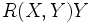$R(X,Y)Y$ and$X$ with respect to$g$.

Divide this by the square of the area of the parallelogram formed by$X$ and$Y$. This ratio defines the sectional curvature of$\Pi$, denoted as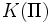$K(\Pi)$.

Here is the more explicit formula:$\frac{g(R(X,Y)Y,X)}{g(X,X)g(Y,Y) - g(X,Y)^2}$

Note that the denominator cannot vanish because$X$ and$Y$ are independent vectors.

For a pseudo-Riemannian manifold

Further information: Sectional curvature for a pseudo-Riemannian manifold

We can also define the sectional curvature of a pseudo-Riemannian manifold. The same definition works.

Facts

Sectional curvature determines Riemann curvature tensor

This is analogous to the fact that Ricci curvature determines the Ricci curvature tensor

The following facts are true about the Riemann curvature tensor:

• The Riemann curvature tensor can be viewed as a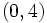$(0,4)$-tensor: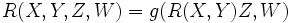$R(X,Y,Z,W) = g(R(X,Y)Z,W)$

• The above map is antisymmetric in$X, Y$ (For full proof, refer: Antisymmetry of Riemann curvature tensor and antisymmetric in$Z$ and$W$. Further it is symmetric in the pairs$(X,Y)$ and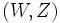$(W,Z)$. Hence, it is a symmetric bilinear form on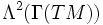$\Lambda^2(\Gamma(TM))$.

Thus, given the sectional curvature for all tangent planes, we can back-calculate the Riemann curvature tensor from it by the usual polarization trick. The idea is to view the Riemann curvature tensor as a symmetric bilinear form on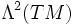$\Lambda^2(TM)$, and the sectional curvature as the values that this form takes on pairs$(\Pi,\Pi)$ of (special kinds of) unit vectors in$\Lambda^2(\Gamma(TM))$.